Question

# A circuit is constructed with five capacitors and a battery as shown. The values for the...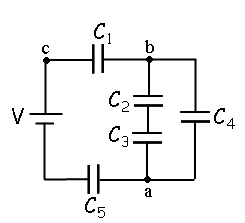A circuit is constructed with five capacitors and a battery as shown. The values for the capacitors are: C1 = C5 = 4.2 μF, C2 = 1 μF, C3 = 5.8 μF, and C4 = 4.4 μF. The battery voltage is V = 12 V.

1)

What is Cab, the equivalent capacitance between points a and b?μF

2)

What is Cac, the equivalent capacitance between points a and c?

3)

What is Q5, the charge on capacitor C5?μC

4)

What is Q2, the charge on C2?

5)

What is Q1, the charge on capacitor C1?μC

6)

What is V4, the voltage across capacitor C4?

C. c ーC4 23 CC 5 IC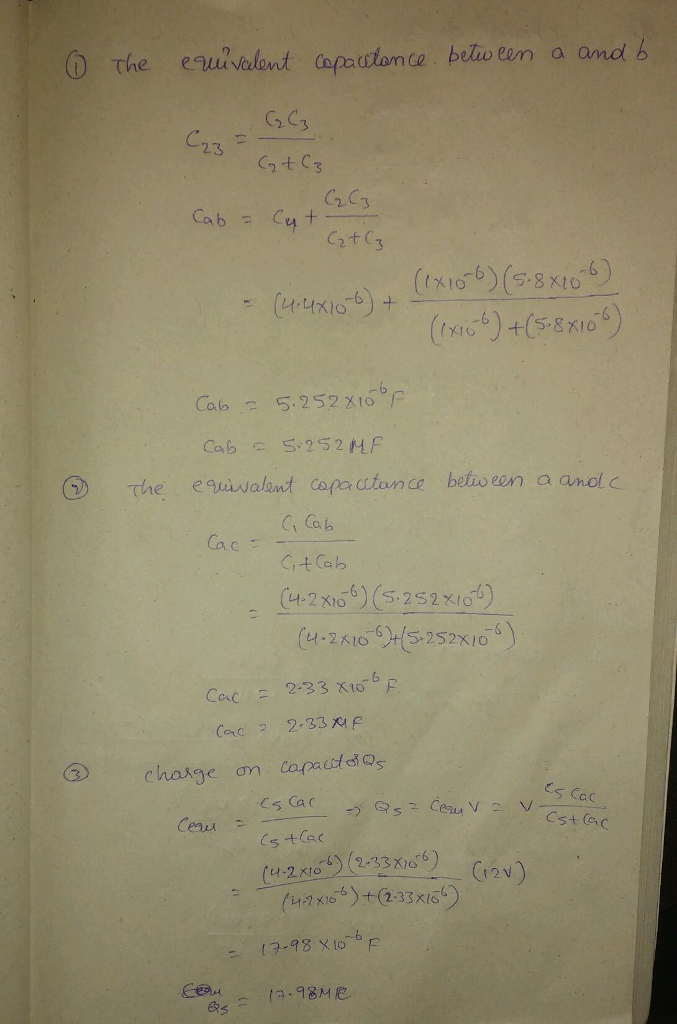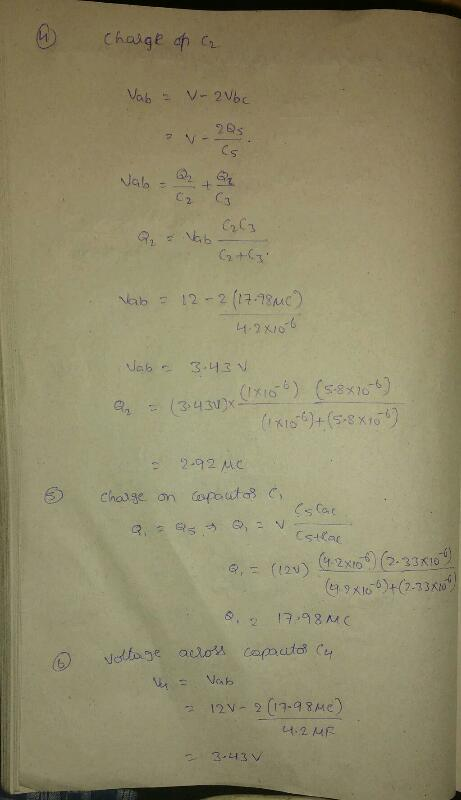#### Earn Coins

Coins can be redeemed for fabulous gifts.

Similar Homework Help Questions
• ### A circuit is constructed with five capacitors and a battery as shown. The values for...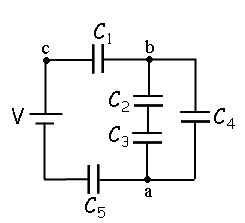A circuit is constructed with five capacitors and a battery as shown. The values for the capacitors are: C1 = C5 = 3.9 μF, C2 = 1.9 μF, C3 = 7.6 μF, and C4 = 2.2 μF. The battery voltage is V = 12 V. 4)What is Q2, the charge on C2? 5)What is Q1, the charge on capacitor C1? C1 b HH Cz C4 Ca HH a C5

• ### A circuit is constructed with five capacitors and a battery as shown. The values for the...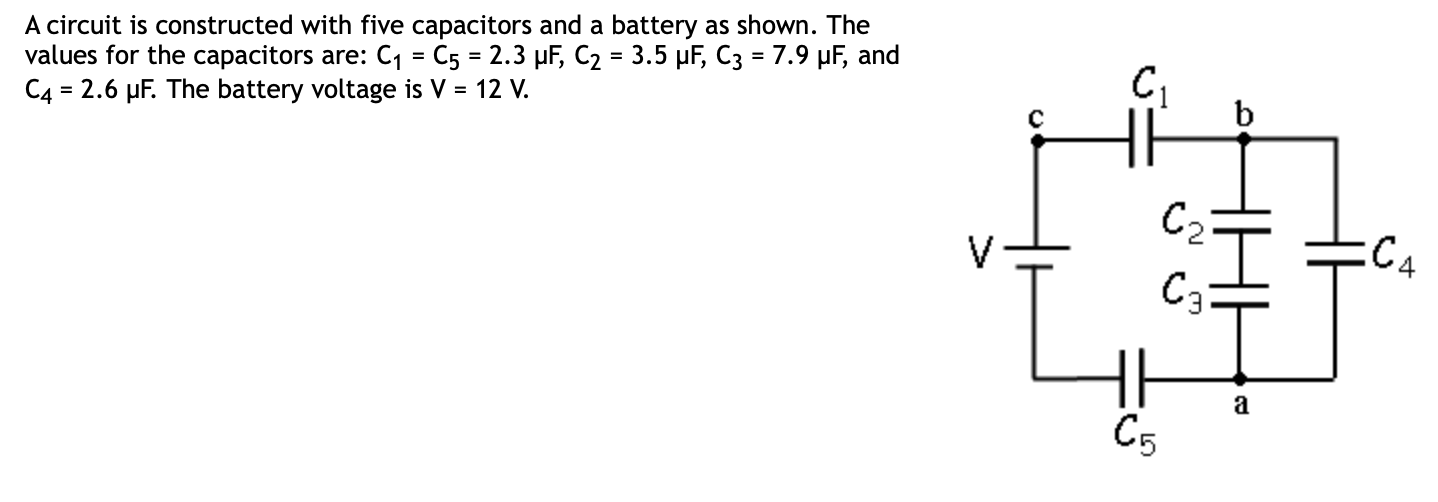A circuit is constructed with five capacitors and a battery as shown. The values for the capacitors are: C1 = C5 = 2.3 uF, C2 = 3.5 uF, C3 = 7.9 pF, and C4 = 2.6 uF. The battery voltage is V = 12 V. EC4 4) What is Q2, the charge on Cz? C Submit 5) What is Q1, the charge on capacitor Cy? uc Submit 6) What is V4, the voltage across capacitor C4? Submit

• ### Four capacitors are arranged in the circuit shown in the figure. The capacitors have the values...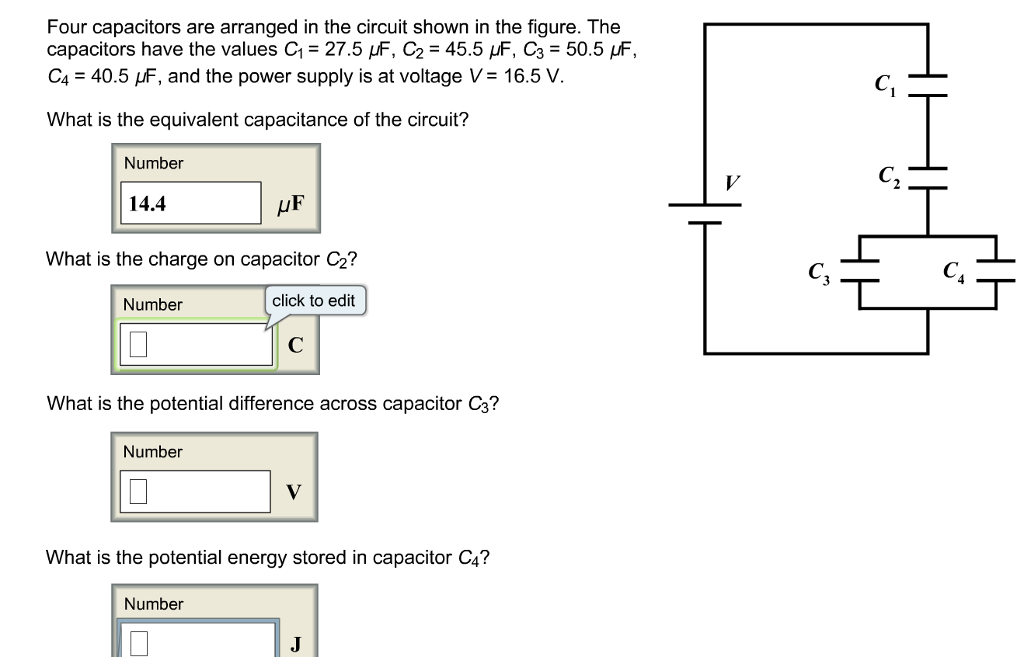Four capacitors are arranged in the circuit shown in the figure. The capacitors have the values C1 = 27.5 μF, C2 = 45.5 μF, C3 = 50.5 μF, C4 = 40.5 μF, and the power supply is at voltage V = 16.5 V. What is the equivalent capacitance of the circuit? Four capacitors are arranged in the circuit shown in the figure. The capacitors have the values C1-27.5 μF, C2 = 45.5 μF, C3 = 50.5 F, C.-40.5 and the...

• ### Vbat = C4 C5 Consider the circuit shown above. The battery provides a voltage of Vbat...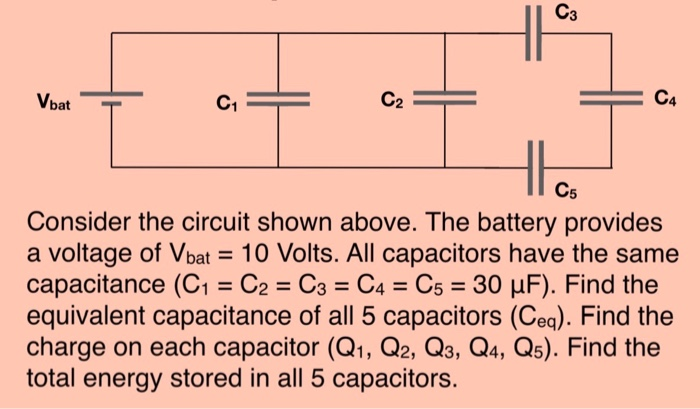Vbat = C4 C5 Consider the circuit shown above. The battery provides a voltage of Vbat = 10 Volts. All capacitors have the same capacitance (C1 = C2 = C3 = C4 = C5 = 30 uF). Find the equivalent capacitance of all 5 capacitors (Ceg). Find the charge on each capacitor (Q1, Q2, Q3, Q4, Q5). Find the total energy stored in all 5 capacitors.

• ### Q22 (Capacitors) The figure below shows a 15.0 V battery and four uncharged capacitors of capacitances...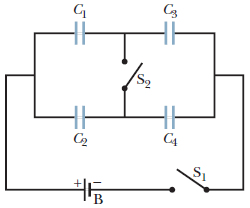Q22 (Capacitors) The figure below shows a 15.0 V battery and four uncharged capacitors of capacitances C1 = 8.00 μF, C2 = 4.00 μF, C3 = 6.00 μF, and C4 = 3.00 μF. If only switch S1 is closed, what is the charge on each capacitor? (a)    C1 __________ μC (b)    C2 __________ μC (c)    C3 __________μC (d)    C4 __________ μC If both switches are closed, what is the charge on each capacitor? (e)    C1 __________μC (f )    C2 __________μC (g)    C3 __________ μC (h)    C4 __________ μC...

• ### A circuit is constructed with five capacitors and a battery as shown. The values for the...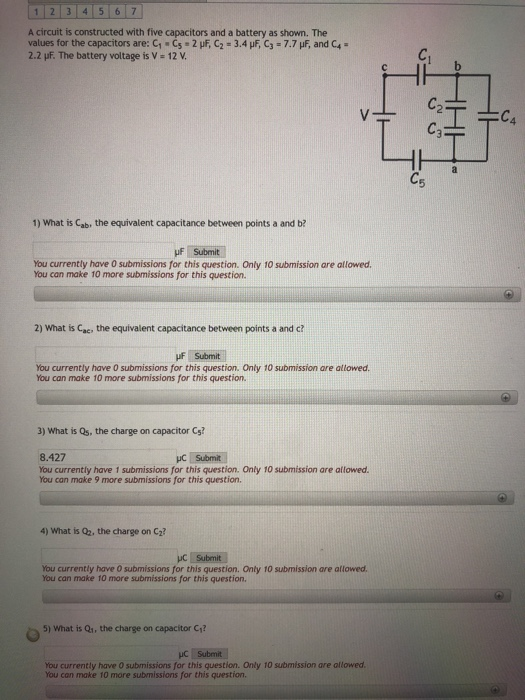A circuit is constructed with five capacitors and a battery as shown. The values for the capacitors are: C1 . С5 . 2 ,F, c,. 3.4 F, C3-77 F, and C+" 2.2 pF. The battery voltage is V 12v 1) What is Cab, the equivalent capacitance between points a and b? uF Submit You currently have O submissions for this question. Only 10 submission are allowed. You can make 10 more submissions for this question. 2) What is ac, the...

• ### Consider the circuit as shown. The power supply has a voltage V = 34 V and...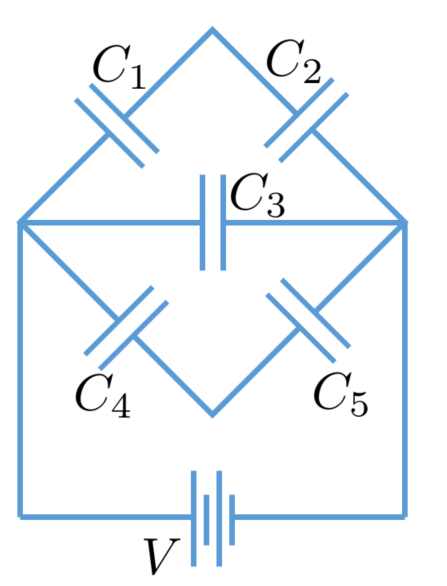Consider the circuit as shown. The power supply has a voltage V = 34 V and the capacitors have capacitance C1 = C2 = C3 = C4 = 80 µF and C5 = 208 µF. (a) What is the equivalent capacitance of this circuit? (b) What is the charge on capacitor C3? (c) What is the voltage on capacitor C3? (d) What is the charge on capacitor C5? (e) What is the voltage on capacitor C5?

• ### The figure shows a circuit with an ideal battery and set of capacitors. The battery has...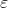The figure shows a circuit with an ideal battery and set of capacitors. The battery has an emf =12 V. The capacitors have capacitances C1 = C2 = C3 = C4 = 6 nF. (a) What is the equivalent capacitance of the entire network of capacitors? (b) What is the electrical energy stored in the capacitor with capacitance C2? We were unable to transcribe this imageProblem 6 C. The figure shows a circuit with an ideal battery and set of...

• ### In the figure a 27 V battery is connected across capacitors of capacitances C1-C6-3.0 μF and...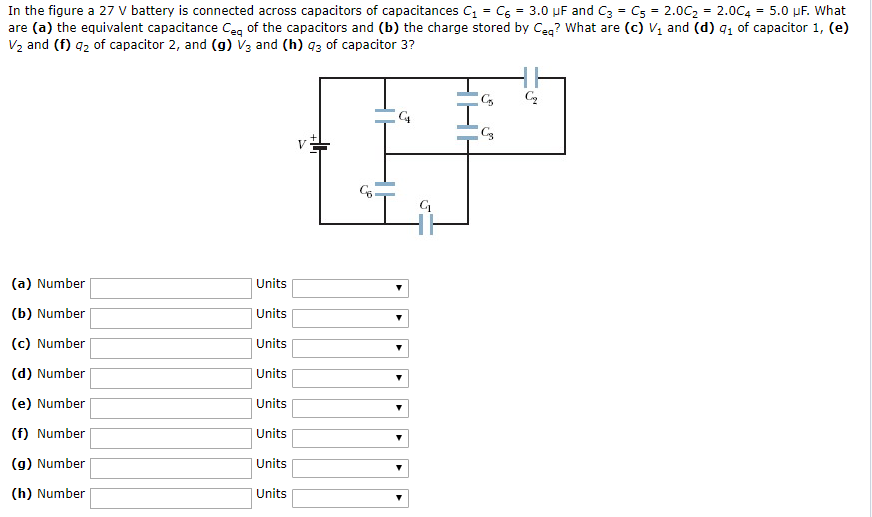In the figure a 27 V battery is connected across capacitors of capacitances C1-C6-3.0 μF and C3-Cs* 2.0C,-2.0C4-5.0 μF, what are (a) the equivalent capacitance Ceq of the capacitors and (b) the charge stored by Ceq? What are (c) V1 and (d) q1 of capacitor 1, (e) V2 and (f) 2 of capacitor 2, and (g) V3 and (h) 3 of capacitor 3? Gs C2 C4 CS C5 (a) Number (b) Number (c) Number (d) Number (e) Number (f) Number...